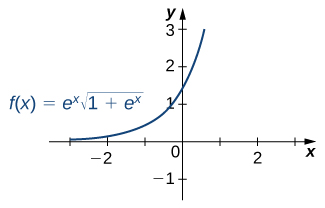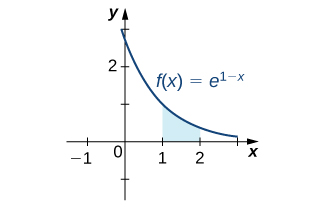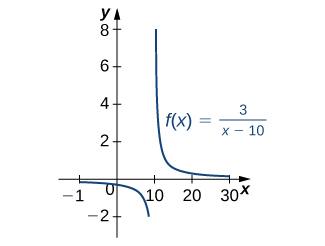In Progress
Lesson 22 of 24
In Progress

# Integrals Involving Exponential and Logarithmic Functions

Exponential and logarithmic functions are used to model population growth, cell growth, and financial growth, as well as depreciation, radioactive decay, and resource consumption, to name only a few applications. In this section, we explore integration involving exponential and logarithmic functions.

# Integrals of Exponential Functions

The exponential function is perhaps the most efficient function in terms of the operations of calculus. The exponential function,is its own derivative and its own integral.

### Rule: Integrals of Exponential Functions

Exponential functions can be integrated using the following formulas.

### Finding an Antiderivative of an Exponential Function

Find the antiderivative of the exponential function.

#### Solution

Use substitution, settingand thenMultiply the du equation by −1, so you now haveThen,

Find the antiderivative of the function using substitution:#### Hint

Letequal the exponent on.

A common mistake when dealing with exponential expressions is treating the exponent onthe same way we treat exponents in polynomial expressions. We cannot use the power rule for the exponent on. This can be especially confusing when we have both exponentials and polynomials in the same expression, as in the previous checkpoint. In these cases, we should always double-check to make sure we’re using the right rules for the functions we’re integrating.

### Square Root of an Exponential Function

Find the antiderivative of the exponential function#### Solution

First rewrite the problem using a rational exponent:

Using substitution, chooseThen,We have ((Figure))

ThenFigure 1. The graph shows an exponential function times the square root of an exponential function.

Find the antiderivative of#### Hint

Let### Using Substitution with an Exponential Function

Use substitution to evaluate the indefinite integral#### Solution

Here we choose to letequal the expression in the exponent on. LetandAgain, du is off by a constant multiplier; the original function contains a factor of 32, not 62. Multiply both sides of the equation byso that the integrand inequals the integrand in. Thus,

Integrate the expression inand then substitute the original expression inback into theintegral:

Evaluate the indefinite integral#### Hint

LetAs mentioned at the beginning of this section, exponential functions are used in many real-life applications. The numberis often associated with compounded or accelerating growth, as we have seen in earlier sections about the derivative. Although the derivative represents a rate of change or a growth rate, the integral represents the total change or the total growth. Let’s look at an example in which integration of an exponential function solves a common business application.

A price–demand function tells us the relationship between the quantity of a product demanded and the price of the product. In general, price decreases as quantity demanded increases. The marginal price–demand function is the derivative of the price–demand function and it tells us how fast the price changes at a given level of production. These functions are used in business to determine the price–elasticity of demand, and to help companies determine whether changing production levels would be profitable.

### Finding a Price–Demand Equation

Find the price–demand equation for a particular brand of toothpaste at a supermarket chain when the demand is 50 tubes per week at $2.35 per tube, given that the marginal price—demand function,fornumber of tubes per week, is given as If the supermarket chain sells 100 tubes per week, what price should it set? #### Solution To find the price–demand equation, integrate the marginal price–demand function. First find the antiderivative, then look at the particulars. Thus, Using substitution, letandThen, divide both sides of the du equation by −0.01. This gives The next step is to solve for C. We know that when the price is$2.35 per tube, the demand is 50 tubes per week. This means

Now, just solve for C:

Thus,

If the supermarket sells 100 tubes of toothpaste per week, the price would be

The supermarket should charge \$1.99 per tube if it is selling 100 tubes per week.

### Evaluating a Definite Integral Involving an Exponential Function

Evaluate the definite integral#### Solution

Again, substitution is the method to use. LetsoorThenNext, change the limits of integration. Using the equationwe have

The integral then becomes

See (Figure).Figure 2. The indicated area can be calculated by evaluating a definite integral using substitution.

Evaluate#### Hint

Let### Growth of Bacteria in a Culture

Suppose the rate of growth of bacteria in a Petri dish is given bywhereis given in hours andis given in thousands of bacteria per hour. If a culture starts with 10,000 bacteria, find a functionthat gives the number of bacteria in the Petri dish at any time. How many bacteria are in the dish after 2 hours?

#### Solution

We have

Then, atwe havesoand we get

At timewe have

After 2 hours, there are 17,282 bacteria in the dish.

From (Figure), suppose the bacteria grow at a rate ofAssume the culture still starts with 10,000 bacteria. FindHow many bacteria are in the dish after 3 hours?

#### SolutionThere are 20,099 bacteria in the dish after 3 hours.

#### Hint

Use the procedure from (Figure) to solve the problem.

### Fruit Fly Population Growth

Suppose a population of fruit flies increases at a rate ofin flies per day. If the initial population of fruit flies is 100 flies, how many flies are in the population after 10 days?

#### Solution

Letrepresent the number of flies in the population at time. Applying the net change theorem, we have

There are 122 flies in the population after 10 days.

Suppose the rate of growth of the fly population is given byand the initial fly population is 100 flies. How many flies are in the population after 15 days?

#### Solution

There are 116 flies.

#### Hint

Use the process from (Figure) to solve the problem.

### Evaluating a Definite Integral Using Substitution

Evaluate the definite integral using substitution:#### Solution

This problem requires some rewriting to simplify applying the properties. First, rewrite the exponent onas a power of, then bring the2 in the denominator up to the numerator using a negative exponent. We have

Letthe exponent on. Then

Bringing the negative sign outside the integral sign, the problem now reads

Next, change the limits of integration:

Notice that now the limits begin with the larger number, meaning we must multiply by −1 and interchange the limits. Thus,

Evaluate the definite integral using substitution:#### Hint

Let# Integrals Involving Logarithmic Functions

Integrating functions of the formresult in the absolute value of the natural log function, as shown in the following rule. Integral formulas for other logarithmic functions, such asandare also included in the rule.

### Rule: Integration Formulas Involving Logarithmic Functions

The following formulas can be used to evaluate integrals involving logarithmic functions.

### Finding an Antiderivative InvolvingFind the antiderivative of the function#### Solution

First factor the 3 outside the integral symbol. Then use the−1 rule. Thus,

See (Figure).Figure 3. The domain of this function is

Find the antiderivative of#### Hint

Follow the pattern from (Figure) to solve the problem.

### Finding an Antiderivative of a Rational Function

Find the antiderivative ofThis can be rewritten asUse substitution. LetthenAlter du by factoring out the 2. Thus,

Rewrite the integrand in:

Then we have

### Finding an Antiderivative of a Logarithmic Function

Find the antiderivative of the log function#### Solution

Follow the format in the formula listed in the rule on integration formulas involving logarithmic functions. Based on this format, we have

Find the antiderivative of#### Hint

Follow (Figure) and refer to the rule on integration formulas involving logarithmic functions.

(Figure) is a definite integral of a trigonometric function. With trigonometric functions, we often have to apply a trigonometric property or an identity before we can move forward. Finding the right form of the integrand is usually the key to a smooth integration.

### Evaluating a Definite Integral

Find the definite integral of#### Solution

We need substitution to evaluate this problem. LetsoRewrite the integral in terms of, changing the limits of integration as well. Thus,

Then

### Key Concepts

• Exponential and logarithmic functions arise in many real-world applications, especially those involving growth and decay.
• Substitution is often used to evaluate integrals involving exponential functions or logarithms.

# Key Equations

• Integrals of Exponential Functions• Integration Formulas Involving Logarithmic Functions